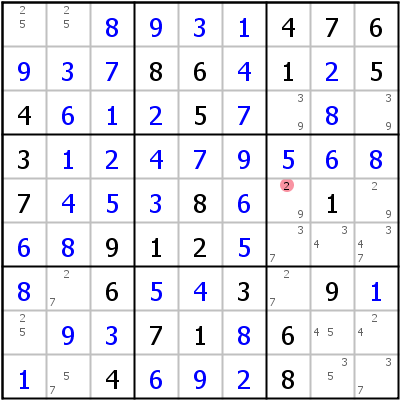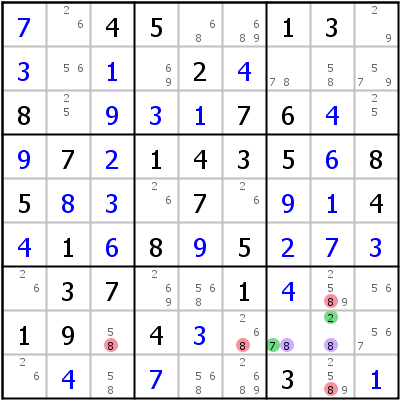Mirrored from Sudopedia, the Free Sudoku Reference Guide

# Avoidable Rectangle

An Unavoidable Rectangle is a 4-cell deadly pattern in a Sudoku solution; for example:

```xy .  .  |  yx .  .  |  .  .  .
yx .  .  |  xy .  .  |  .  .  .
.  .  .  |  .  .  .  |  .  .  .
```

where x and y represent different values, and where either all of the left-hand values are true or all of the right-hand values are true. For this pattern to appear in the solution of a Sudoku with a unique solution, at least one of the four cells in the pattern must be supplied as an initial clue to avoid the rectangle being deadly. Hence the term Unavoidable Rectangle--the designer of the Sudoku cannot avoid providing a given clue in at least one of the cells of the rectangle.

From the solver's standpoint, if we know that the Sudoku has a unique solution, and none of the four cells in the rectangle was supplied as a given, then the pattern described above would indeed be deadly and must be avoided. Hence where none of the rectangle cells is a given, this is an Avoidable Rectangle--there are restrictions on candidate placement if we are to avoid the deadly pattern. The originator of this technique called the pattern an Almost Unavoidable Rectangle, or AUR, but that term was already in use for Almost Unique Rectangles. In his book The Logic of Sudoku, Andrew C Stuart uses the term Avoidable Rectangle, which is how it will be referred to here, especially as it better describes what is going on from the solver's (as opposed to the puzzle designer's) point of view.

Where all four cells in the rectangle are unsolved, the Uniqueness Test strategies can be used to evaluate the situation. This section discusses Avoidable Rectangles involving two or more solved cells--cells whose values are known but that were not provided as initial givens to the Sudoku. There are three kinds of Avoidable Rectangles: Type 1, Type 2, and Type 3; these correspond directly to the Type 1, 2, and 3 Unique Rectangles involved in the Uniqueness Test strategies.

The cells that participate in an Avoidable Rectangle must share exactly two rows, two columns, and two boxes.

## Floor and Ceiling

All of the Avoidable Rectangle patterns have two cells that share a row or column and both of which are solved. These two cells form the floor of the Avoidable Rectangle. The other two cells are called the ceiling. The ceiling contains the cell(s) of the rectangle that have more than one candidate. In the following discussions, the floor cells are designated A, with solved value a, and B, with solved value b. The ceiling cell that shares a row or column with A is designated C and the ceiling cell that shares a row or column with B is designated D. A and D thus are in opposite corners of the rectangle, as are B and C. For example:

```1  .  .  |  23 .  .  |  .  .  .
2  .  .  |  13 .  .  |  .  .  .
.  .  .  |  .  .  .  |  .  .  .
```

Here the leftmost column is the floor and the rightmost column the ceiling. Another example:

```1  .  .  |  2  .  .  |  .  .  .
.  .  .  |  .  .  .  |  .  .  .
23 .  .  |  1  .  .  |  .  .  .
```

Here the top row is the floor and the bottom row is the ceiling.

## Type 1 Avoidable Rectangle

Cell C has the solved value b. Cell D is unsolved and has candidates ax where x is one or more candidate values besides a. If cell D had the value a, the solution would contain the Unavoidable Rectangle pattern, but we know that can't be the case because none of the rectangle cells was a given. Thus we can discard a as a candidate in cell D.

Note the similarity of this strategy to a Type 1 Unique Rectangle.

In the example below r4c37,r5c37 form an Avoidable Rectangle for candidates 2 and 5. 2 can be eliminated from r5c7.## Type 2 Avoidable Rectangle

Cell C has exactly two candidate values bc, and cell D has exactly two candidate values ac. Candidate c must be in one of the ceiling cells or else the solution would have an Unavoidable Rectangle, and we know that is not true because none of the rectangle cells was a given. We can therefore discard candidate c from any cell that shares a house with both C and D.

Note the similarity of this strategy to a Type 2 Unique Rectangle.

In the example below r6c78,r8c78 form the Avoidable Rectangle for candidates 2 and 7 with the additional candidate 8 in r8c78. 8 can therefore be eliminated from r7c8, r9c8, r8c3 and r8c6.## Type 3 Avoidable Rectangle

Cell C has candidates bx and cell D has candidates ay where x and y are one or more additional candidates. A candidate value may appear in both x and y. At least one of the x or y values must occur in the ceiling cells or else we have an Unavoidable Rectangle in the solution, which we know is impossible because none of the rectangle cells was a given. We can therefore treat the ceiling cells as a single pseudo-cell that has all of the x and y values as candidates and use it to form a Naked Subset with other cells that share a house with both ceiling cells. The candidate values for the Naked Subset can then be eliminated from any other cell that shares a house with all cells of the subset.

Note the similarity of this strategy to a Type 3 Unique Rectangle.